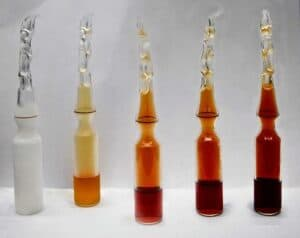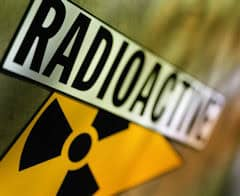## ModelingIn this activity students study an existing model of an irreversible reaction of N2O4 to NO2 and expand it in order to create a chemical equilibrium. In order to teach students to critically assess a model, the concentration quotient has to be calculated at some point in the activity. This activity focusses on the essence of modelling and less on the workings of an equilibrium.In this activity students explore radioactive decay via a given decay model. Based on the model they determine the function, which describes the radioactive decay. Further they modify the model to describe a decay of a real radioactive isotope e.g. Barium 137m and compare how well the model fits the experimental results.Our defense system is not an easy topic to understand. This model helps students to understand the topic more easily. Students can study the impact of factors such as incubation period and infection on the course of an Ebola virus infection. Through inquiery based learning students learn the basics of a viral infection and important factors.